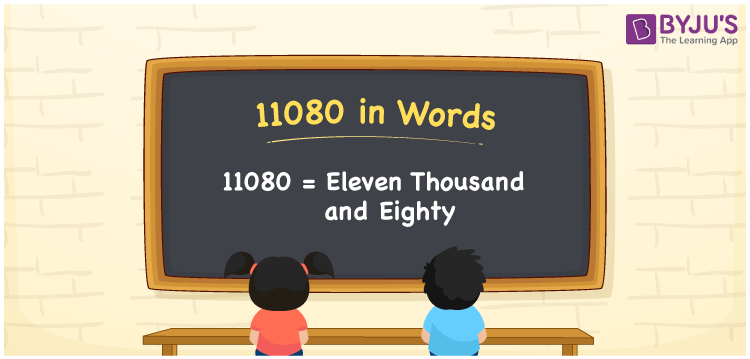# 11080 in Words

11080 in words is written as Eleven thousand eighty. In both the International System of Numerals and the Indian System of Numerals, 11080 is written as Eleven thousand eighty. The number 11080 is a Cardinal Number as it represents some quantity. For example, “that mobile phone costs 11080 rupees”.

 11080 in Words Eleven thousand eighty Eleven thousand eighty in Number 11080

## 11080 in English Words

11080 in English words is read as “Eleven thousand eighty”## How to Write 11080 in Words?

To write 11080 in words, we shall use the place value chart. In the place value chart, put 1 in the ten thousands and thousands, 0 in the hundreds, 8 in the tens and 0 in the ones, respectively. Let us make a place value chart to write the number 11080 in words.

 Ten Thousands Thousands Hundreds Tens Ones 1 1 0 8 0

Thus, we can write the expanded form as

1 × Ten Thousand + 1 × Thousand + 0 × Hundred + 8 × Ten + 0 × One

= 1 × 10000 + 1 × 1000 + 0 × 100 + 8 × 10 + 0 × 1

= 10000 + 1000 + 0 +80 + 0

= 11080

= Eleven thousand eighty.

11080 is a natural number, the successor of 11079 and the predecessor of 11081.

11080 in words – Eleven thousand eighty

• Is 11080 an odd number? – No
• Is 11080 an even number? – Yes
• Is 11080 a perfect square number? – No
• Is 11080 a perfect cube number? – No
• Is 11080 a prime number? – No
• Is 11080 a composite number? – Yes

## Frequently Asked Questions on 11080 in Words

Q1

### How to write 11080 in words?

11080 in words is written as Eleven thousand eighty.
Q2

### How to write 11080 in the International and Indian System of Numerals?

In both, the system of numerals, 11080 in words, is written as Eleven thousand eighty.
Q3

### How to write 11080 in a place value chart?

In the place value chart, write 1 in the ten thousands and thousands, 0 in the hundreds, 8 in the tens and 0 in the ones, respectively.